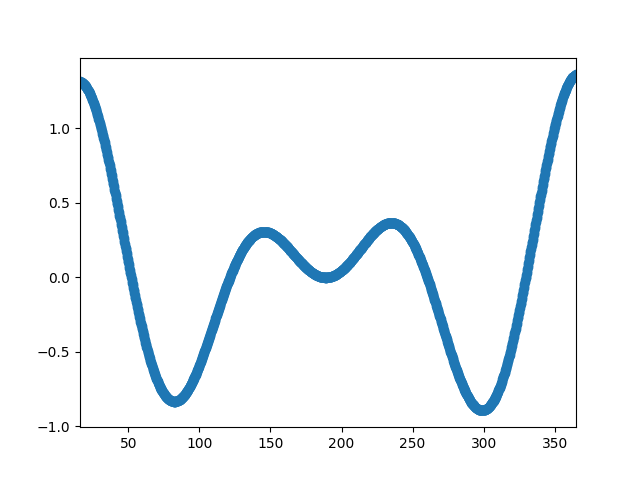Travis-CI:Resampling Data¶

Downsampling lowers the sample rate or sample size of a signal. In this tutorial, the signal is downsampled when the plot is adjusted through dragging and zooming.```import numpy as np
import matplotlib.pyplot as plt

# A class that will downsample the data and recompute when zoomed.
def __init__(self, xdata, ydata):
self.origYData = ydata
self.origXData = xdata
self.max_points = 50
self.delta = xdata[-1] - xdata

def downsample(self, xstart, xend):
# get the points in the view range
mask = (self.origXData > xstart) & (self.origXData < xend)
# dilate the mask by one to catch the points just outside
# of the view range to not truncate the line
# sort out how many points to drop
ratio = max(np.sum(mask) // self.max_points, 1)

# downsample data
xdata = xdata[::ratio]
ydata = ydata[::ratio]

print("using {} of {} visible points".format(

return xdata, ydata

def update(self, ax):
# Update the line
lims = ax.viewLim
if np.abs(lims.width - self.delta) > 1e-8:
self.delta = lims.width
xstart, xend = lims.intervalx
self.line.set_data(*self.downsample(xstart, xend))
ax.figure.canvas.draw_idle()

# Create a signal
xdata = np.linspace(16, 365, (365-16)*4)
ydata = np.sin(2*np.pi*xdata/153) + np.cos(2*np.pi*xdata/127)

fig, ax = plt.subplots()

# Hook up the line
d.line, = ax.plot(xdata, ydata, 'o-')
ax.set_autoscale_on(False)  # Otherwise, infinite loop

# Connect for changing the view limits
ax.callbacks.connect('xlim_changed', d.update)
ax.set_xlim(16, 365)
plt.show()
```

Total running time of the script: ( 0 minutes 0.017 seconds)

Gallery generated by Sphinx-Gallery